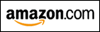﻿ Mixed Integration Practice
INTEGRATION QUIZ #4

NAU STUDENTS:
This exercise gives you new problems to practice—ones requiring integration by parts.
Your in-class quiz will cover all the problems from the first three integration quizzes, together with these new problems.

In this exercise, all functions are assumed to have the required properties for a particular situation.
For example, any function in a denominator is assumed to be nonzero, any function inside a logarithm is assumed to be positive, and so on.

## INTEGRATION BY PARTS

Integration by parts is the integration counterpart to the product rule for differentiation.

Let $u$ and $v$ both be functions of $x$, so that: $$\frac{d}{dx} uv = u\frac{dv}{dx} + v\frac{du}{dx}$$ Integrating both sides with respect to $\,x\,$ gives: $$\int \frac{d}{dx} uv\ dx = \int u\frac{dv}{dx}\ dx + \int v\frac{du}{dx}\ dx$$ This simplifies to: $$uv = \int u\,dv + \int v\,du$$ A bit of rearrangement gives the INTEGRATION BY PARTS FORMULA:

$$\int u\,dv = uv - \int v\,du$$

Here are some observations regarding this formula:
• Can't solve an integral by prior considerations? Then, try parts.
• You must choose $\,u\,$ and $\,dv\$:
• $u$ is differentiated to find $\,du\,$
• $\,dv\,$ is integrated to find $\,v\$ (usually, the constant of integration is chosen to be zero)
• Choose something for $\,dv\,$ that you know how to integrate (else you can't find $\,v\,$, and hence can't use the parts formula).
• More than one choice for $\,dv\,$? Then, choose something for $\,u\,$ that gets simpler when you integrate it.

### EXAMPLE:

The classic problem that requires integration by parts is integrating the natural logarithm function.

Let $\,dv = dx\,$, so that $\,v = x\,$.
Let $u = \ln x\,$, so that $\,du = \frac 1x\,dx\,$.
Then,
$\int \overbrace{\ln x}^{u}\ \overbrace{\ dx\ }^{dv} = \overbrace{(\ln x)}^{u}\cdot\overbrace{\ \ x\ \strut\ }^{v} - \int \overbrace{\strut\ \ x\ \ }^{v}\cdot\overbrace{\frac{1}{x}\,dx}^{du} = x\ln x - \int (1)\,dx = x\ln x - x + C$

### EXAMPLE:

Let $u = x\,$, so that $\,du = \,dx\,$.
Let $\,dv = {\text{e}}^x\,dx\,$, so that $\,v = {\text{e}}^x\,$.
Then,

$\int x{\text{e}}^x\,dx = x{\text{e}}^x - \int {\text{e}}^x\,dx = x{\text{e}}^x - {\text{e}}^x + C$
Master the ideas from this section
by practicing the exercise at the bottom of this page.

On this exercise, you will not key in your answer.
However, you can check to see if your answer is correct.Is this web exercise helping you? I'm spending many hours creating it. Please help me to help you... just watch my 15-second video to see how easy it is to support my work, at absolutely no cost to you! (Really!) Thank you!